# SSC CGL EXAMS 2018 | Quantitative Aptitude Practice Questions (Day-12)

Dear Aspirants, Here we have given the Important SSC Exam 2018 Practice Test Papers. Candidates those who are preparing for SSC 2018 can practice these questions to get more confidence to Crack SSC 2018 Examination.

[WpProQuiz 4294]

1) Rs. 800 becomes Rs. 956 in 3 years at a certain rate of simple interest. If the rate of interest is increased by 4%, what amount will Rs. 800 become in 3 years?

a) Rs. 1010

b) Rs. 1020

c) Rs. 1052

2) The compound interest on Rs. 20,000 at 5% per annum is Rs. 3152.50. The period (in years) is:

a) 1

b) 21/2

c) 3

d) None of these

3) If x/y = z/w then xy + zw = ?

a) (x2 + zb) (y2 + wb)

b) x2y2 + z2 w2

c) x2 w2 + y2 z2

d) (x2 + wb) (y2 + zb)

4) If (x + y): (x–y) = 3 : 2, Then (x2 – yb): (x2 + yb) =?

a) 12 : 11

b) 12 : 13

c) 11 : 13

d) 10 : 13

5) A bag contains Re. 1, 50-p, 25-p coins in the ratio 1 : 2 : 4. If there is Rs. 30 in all, how many Re. 1 coins are there?

a) 10

b) 20

c) 5

d) 15

6) Rs. 864 is divided among A, B and C such that 8 times A’s share is equal to 12 times B’s share and also equal to 6 times C,s share. How much did B get?

a) Rs. 399

b) Rs. 192

c) Rs. 288

d) Rs. 72

7) Two pipes, P and Q can fill a cistern in 12 and 15 minutes, respectively. If both are opened together and at the end of 3 minutes, the first is closed, how much longer will be cistern take to fill?

a) 5 minutes

b) 7 1/2 minutes

c) 8 1/4 minutes

d) 6 3/4 minutes

8) Madhu takes half the time taken by Neelam to go from Bombay to Pune. Neelam take 20 hours to go for the same journey. What is the speed of Neelam if the speed of Madhu is 20 kmph?

a) 20 kmph

b) 10 kmph

c) 15 kmph

d) None of these

9) Trisha goes to office at 10 kmph and reaches the office 2 minutes late. If she goes at 15 kmph she reaches the office 2 minutes earlier than the scheduled time. What is the distance of her office?

a) 3 km

b) 2 km

c) 4 km

d) 2.5 km

10) A worker reaches his factory 3 minutes late of his speed from his house to the factory is 5 kmph. If he walks at a speed of 6 kmph, he reaches the factory 7 minutes early. The distance of the factory from his house is:

a) 3 km

b) 4 km

c) 5 km

d) 6 km

11) A man covers 12 km upstream and 18 km downstream in 3 hours, while he covers 36 km upstream and 24 km downstream in 6.5 hours. What is the speed of the current (in kmph):

a) 1

b) 1.5

c) 2

d) 2.5

12) The ratio of speed of a boat to that of the current of water is 36 : 5. The boat goes along with the current in 5 hours 10 minutes. It will come back in:

a) 5 hrs 20 min

b) 6 hrs 10 min

c) 6 hrs 50 min

d) 7 hrs 10 min

13) AB is a chord of a circle of radius 14 cm. The chord subtends a right angle at the centre of the circle. Find the area of the minor segment.

a) 98 sq cm

b) 56 sq cm

c) 112 sq cm

d) None of these

14) A cone and a hemisphere have equal bases and equal volumes. Find the ratio of their heights.

a) 1 : 2

b) 2 : 1

c) 3 : 1

d) None of these

15) Find the area of the quadrilateral ABCD. (Given, √3 = 1.73)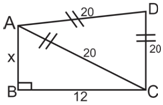a) 452 sq units

b) 269 sq units

c) 134.5 sq units

d) 1445 g cm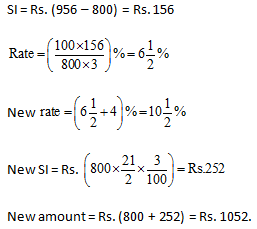Interest for first year = 5% of 20000 = Rs. 1000

Interest for second year = 5% of 21000 = Rs. 1050

Interest for third year = 5% of 22050 = Rs. 1102.50

Thus, the total interest earned in 3 yrs = 1000 + 1050 + 1102.50 = Rs. 3152.50.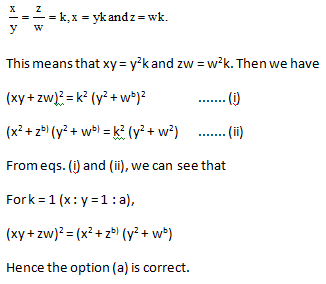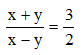or 2x + 2y = 3x – 3y

or x = 5y

or x : y = 5 : 1

Let x = 5k and y = k

(x2 – yb) : (x2 + yb) = (25 k2 – kb) : (25k2 + kb)

= 24 k2 : 26 k2 = 12 : 13.

Let the number of Re. 1, 50-paise, 25 paisa coins are x, 2x, 4x.

Total value of all the coins = 1×x + 0.50×2x + 0.25×4x = 3x

or 3x = 30.

Thus, x = 10.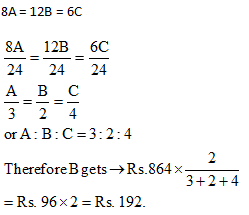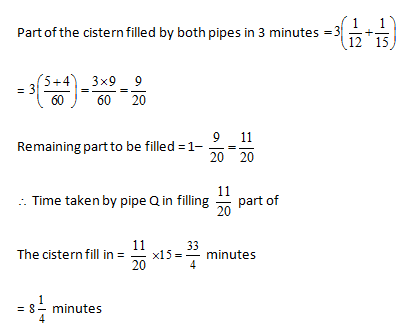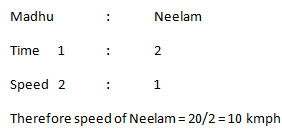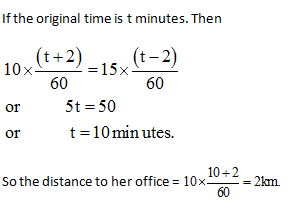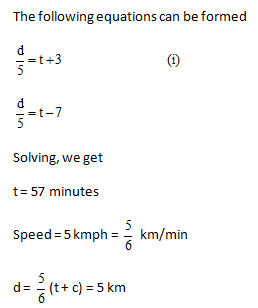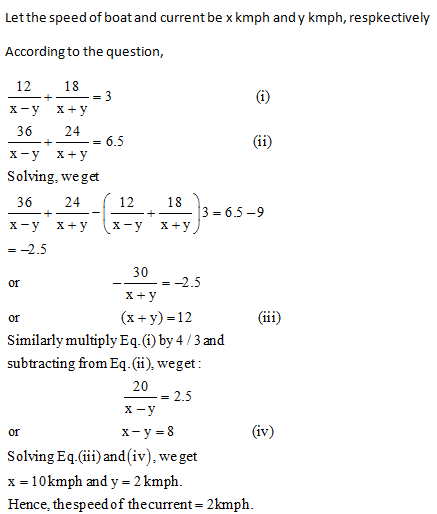Let the speed of the boat and of the current be 36v and 5v (in km per minutes), respectively.

Total distance covered by the boat in downstream = 41v  310 km

Let the boat takes t minutes to come back,

Then

41v × 310 = (36v –5v)t

t = 410 minutes = 6 hours 50 minutes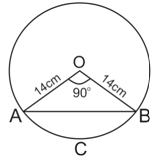Area of the sector ACBO =  90π × 142 / 30

= 154 sq cm

Area of the triangle AOB = (14 × 14) /2 = 98 sq cm

Area of the segment ACB = Area sector

ACBO – Area of the triangle AOB = 56 sq cm

Questions of this type should be solved without the use of pen and paper. A good authority over formulae will make things easier.

Volume of the cone = (πr2 h)/3 = volume of a hemisphere = 2 πr3 / 3

Height of a hemisphere = Radius of its base

So the question s effectively asking us to find out h/r

By the formula above we can easily see that h/r = 2/1.

Area of quadrilateral = Area of right angled triangle + Area of equilateral triangle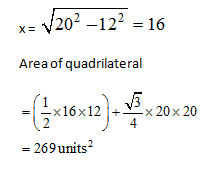### *******************